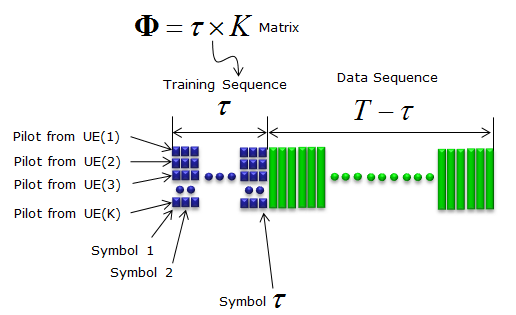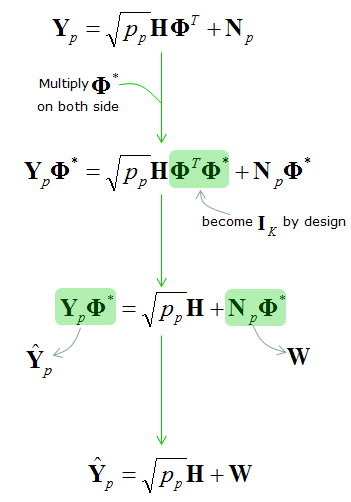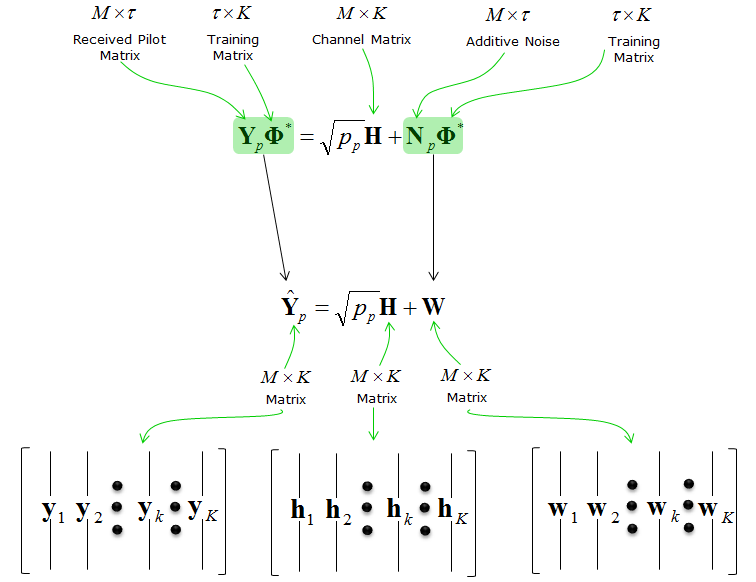5G - Massive MIMO                                                          Home : www.sharetechnote.com Massive MIMO Channel Estimation - Examples   Following is based on Linear Precoding Performance of Massive MU-MIMO Downlink System (  ).  This is to estimate the quality of Uplink channel quality by eNB.  In most of the cases up to LTE, most of channel estimation was done by UE side using the reference signal transmitted by eNB, but in Massive MIMO system it would be too much overhead for UE to do if it has to do channel estimation for such a many antenna and more seriously it would require too much resources for reference signal for downlink signal. So one of the most frequently suggested idea for 5G Massive MIMO is to use TDD and let eNB perform channel estimation using the uplink channel and apply that information to configure downlink signal. This thesis is about this idea meaning Channel Estimation of UL signal.   First, last illustrate overall system to be analyzed in this thesis. I think you are familiar with this kind of drawing by now. You might notice a big difference between this illustration and the other one you saw in many of other channel model page. Can you guess what it is ? It is the direction of the arrow. In most of other channel estimation page, the direction of the arrow were from eNB to UE. But in this case, the direction is from UE to eNB meaning UE antenna is Tx and eNB antenna is Rx.Another important thing to know about channel estimation is to figure out how the reference signal is allocated in transmitted subframe. In this thesis, the reference signal in an uplink subframe is allocated as shown below.With the channel matrix and reference signal, you can write a channel model for this system as shown below. Whenever you see any channel mode (system equation), first check the dimension of each matrix and vectors and try to understand the meaning of each colums and rows of the matrix. Then you would have pretty good understanding of the meaning of the system equation. (In channel Matrix H, the number of rows corresponds to the number of Rx antenna and the number of columns corresponds to the number of Tx antenna).Following is purely mathematical manipulation to simplify the system equation as follows. (This is where you can find the reason why you should learn such a boring subject called Linear Algebra :)Just for clearing up the confusion in your mind, let's briefly check the dimension of each matrix/vector and meaning of them. It can be summarized as below.From the simplified system equation, you can write an equation for the received signal for each antenna as shown below.Assuming the base station is using MMSE channel estimation, each of the channel vector (the vector from one transmitter antenna to all the reciever antenna) can be described as follows. It would be difficult to understand how this can be derived without refering to additional reference. I am just writing the conclusion and let it up to each of the readers to refer to additional references to figure out the details of the derivation. This is the esimated value of each channel path.Reference    Linear Precoding Performance of Massive MU-MIMO Downlink System Eakkanol Pakdeejit Department of Electrical Engineering, Linkopings Universitet